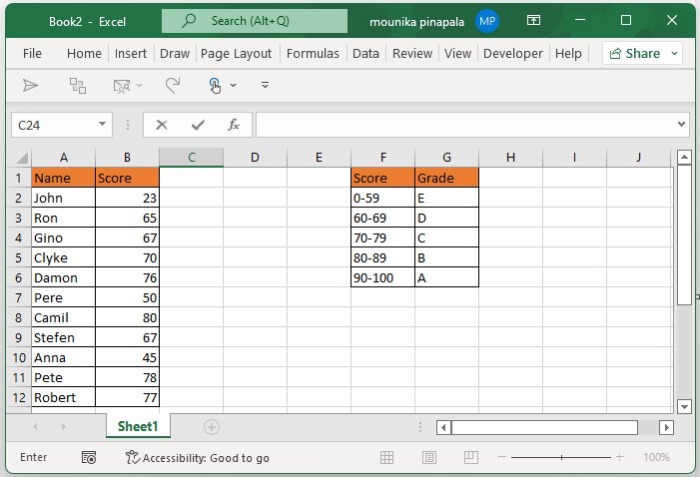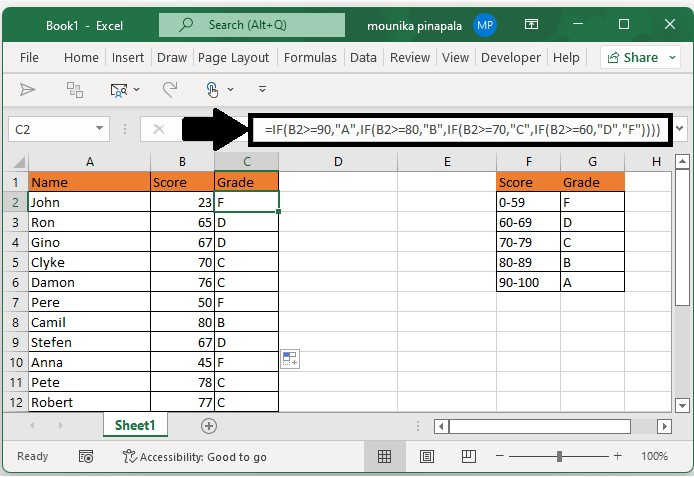# How to Calculate or Assign Letter Grade in Excel

If you need to give the letter grade based on the score values, then the nested IF function in a Microsoft Excel sheet can help you to do this task. Kindly follow the below steps to understand clearly.

## Step 1

Open an excel sheet and enter the data of student names and their score with that enter the Min to Max scores with grades as shown in the below screenshot for your reference.## Step 2

The syntax that we are going to use in this case is given below.

=IF (condition1, value_if_true1, IF (condition2, value_if_true2, IF (condition3, value_if_true3, value_if_false3)))

Note − In the above syntax,

• condition 1, condition 2, and condition 3 are the conditions which you want to check or test.

• value_if_true1, value_if_true2, value_if_true3 are the values which you want to check if the result of the condition is true.

• value_if_false3 is the value that you want to give or return if the result of the condition is false.

## Step 3

Now you need to enter the below given formula in the cell which consists of Grade to calculate the grade and drag the fill handle down till you want to add the formula and calculate the grade as shown in the below screenshot for your reference.

=IF(B2>=90,"A",IF(B2>=80,"B",IF(B2>=70,"C",IF(B2>=60,"D","F"))))Now you see that the grades of the students are determined.

If you observe the above screenshot, you can understand that for students who scored between 90-100 their grade is determined as "A", for students who scored 80-89 their grade is determined as "B", and for students who scored 70-79 their grade is determined as "C", for students who scored 60-69 their grade is determined as "D", students who scored 0-59 their grade is determined as "F".

## Conclusion

In this tutorial, we used a simple example to demonstrate how you can calculate or assign letter grade in Excel.

Updated on: 03-Feb-2023

1K+ Views